Martin McBride, 2017-02-21
Categories numbers

Computers calculate in binary. The basic operations are similar to how you would do arithmetic with a pencil and paper, but of course we must use binary rather than base 10. Once you get your head around it, binary is actually easier than base 10 in some ways.

Here we will look at binary addition.

## The basics

Here are the basic addition results you need to know:The first case are easy. 0 + 0 is 0, and 1 + 0 is 1.

1 + 1 equals 2, of course, but remember that 2 in binary is 10.

Similarly, 1 + 1 + 1 equals 3, but 3 in binary is 11.

To add larger binary numbers, we use a technique which is similar to traditional long addition. For example, here is a 3 digit binary addition: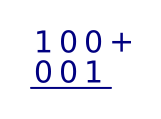We add each column, starting at the right (least lowest place value). In the first column, 0 and 1 give 1.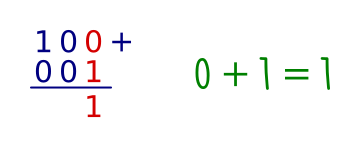In the second column, 0 and 0 give 0.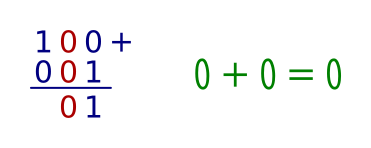In the third column, 1 and 0 give 1.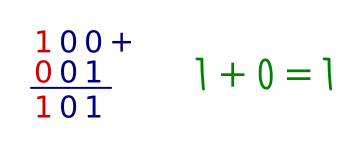We can check the result. Binary 100 is equal to 4, binary 001 is equal to 1. Binary 101 is equal to 5, so the answer is correct.

The previous sum was easy because none of the columns contained two 1s. In this next example, we have to carry digits over:In the first column, 1 and 1 give 10 binary. This means that we put a 0 in the result, but we have 1 to carry over to the next column: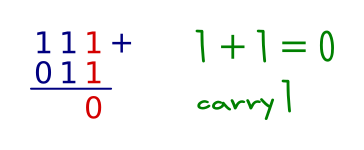In the second column, we have 1 and 1 plus the extra 1 we carried over from before. This gives 11 in binary. So we put a 1 in the result and carry 1 over to the next column: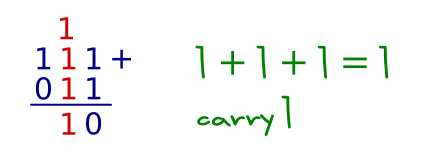In the third column, we have 1 and 0 plus the extra 1 we carried over from before. This gives 10 in binary. So we put a 0 in the result and carry 1 over to the next column: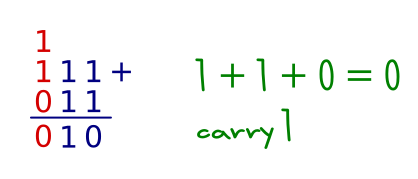We haven't quite finished yet, because we still have the 1 carried over from last time. We create and extra column and put the carry value in that: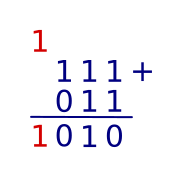Once again, we can check our result. 111 binary is equal to 7, 011 binary is equal to 3, and 1010 binary is equal to 10.